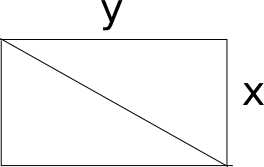Question 16

# Instead of walking along two adjacent sides of a rectangular field, a boy took a short cut along the diagonal and saved a distance equal to half the longer side. Then the ratio of the shorter side to the longer side is

SolutionLet x be the shorter side and y be the longer one. The shortcut route would be of length $$\sqrt{x^2+y^2}$$. According to given condition we know that (x+y)- $$\sqrt{x^2+y^2}$$ = y/2 . Solving, we get 1=(x/y)+(1/4)

=> x : y = 3 : 4. Hence option D is the correct answer.